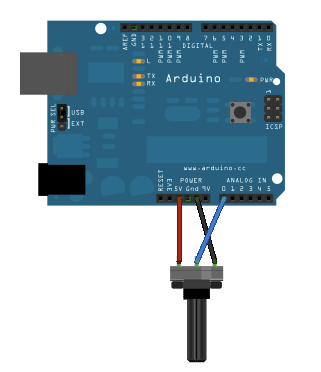This example shows you how to read an analog input on analog pin 0, convert the values from analogRead() into voltage, and print it out to the serial monitor of the Arduino Software (IDE).

### Hardware Required

• Arduino or Genuino Board
• 10k ohm potentiometer

### Circuitimage developed using Fritzing. For more circuit examples, see the Fritzing project page

Connect the three wires from the potentiometer to your board. The first goes to ground from one of the outer pins of the potentiometer. The second goes to 5 volts from the other outer pin of the potentiometer. The third goes from the middle pin of the potentiometer to analog input 0.

By turning the shaft of the potentiometer, you change the amount of resistance on either side of the wiper which is connected to the center pin of the potentiometer. This changes the voltage at the center pin. When the resistance between the center and the side connected to 5 volts is close to zero (and the resistance on the other side is close to 10 kilohms), the voltage at the center pin nears 5 volts. When the resistances are reversed, the voltage at the center pin nears 0 volts, or ground. This voltage is the analog voltage that you're reading as an input.

The microcontroller of the board has a circuit inside called an analog-to-digital converter or ADC that reads this changing voltage and converts it to a number between 0 and 1023. When the shaft is turned all the way in one direction, there are 0 volts going to the pin, and the input value is 0. When the shaft is turned all the way in the opposite direction, there are 5 volts going to the pin and the input value is 1023. In between, analogRead() returns a number between 0 and 1023 that is proportional to the amount of voltage being applied to the pin.

### Code

In the program below, the very first thing that you do will in the setup function is to begin serial communications, at 9600 bits of data per second, between your board and your computer with the line:

`Serial.begin(9600);`

Next, in the main loop of your code, you need to establish a variable to store the resistance value (which will be between 0 and 1023, perfect for an ` int `datatype) coming in from your potentiometer:

` int sensorValue = analogRead(A0);`

To change the values from 0-1023 to a range that corresponds to the voltage the pin is reading, you'll need to create another variable, a `float`, and do a little math. To scale the numbers between 0.0 and 5.0, divide 5.0 by 1023.0 and multiply that by sensorValue :

`float voltage= sensorValue * (5.0 / 1023.0);`

Finally, you need to print this information to your serial window as. You can do this with the command Serial.println() in your last line of code:

` Serial.println(voltage)`

Now, when you open your Serial Monitor in the Arduino IDE (by clicking on the icon on the right side of the top green bar or pressing Ctrl+Shift+M), you should see a steady stream of numbers ranging from 0.0 - 5.0. As you turn the pot, the values will change, corresponding to the voltage coming into pin A0.

/*

Reads an analog input on pin 0, converts it to voltage, and prints the result to the Serial Monitor.
Graphical representation is available using Serial Plotter (Tools > Serial Plotter menu).
Attach the center pin of a potentiometer to pin A0, and the outside pins to +5V and ground.

This example code is in the public domain.

*/

// the setup routine runs once when you press reset:
void setup() {
// initialize serial communication at 9600 bits per second:
Serial.begin(9600);
}

// the loop routine runs over and over again forever:
void loop() {
// read the input on analog pin 0: